# CBSE Class 10 Science Human Eye and Colorful World Assignment Set B

Read and download free pdf of CBSE Class 10 Science Human Eye and Colourful World Assignment Set B. Get printable school Assignments for Class 10 Science. Standard 10 students should practise questions and answers given here for Chapter 11 Human Eyes And Colourful World Science in Grade 10 which will help them to strengthen their understanding of all important topics. Students should also download free pdf of Printable Worksheets for Class 10 Science prepared as per the latest books and syllabus issued by NCERT, CBSE, KVS and do problems daily to score better marks in tests and examinations

## Assignment for Class 10 Science Chapter 11 Human Eyes And Colourful World

Class 10 Science students should refer to the following printable assignment in Pdf for Chapter 11 Human Eyes And Colourful World in standard 10. This test paper with questions and answers for Grade 10 Science will be very useful for exams and help you to score good marks

### Chapter 11 Human Eyes And Colourful World Class 10 Science Assignment

Question : The colored light that refracts most while passing through a prism is
a. Yellow
b. Violet
c. Blue
d. Red

Question : Stars appears to be twinkling because of
a. atmospheric refraction
b. reflection
c. Tyndall effect
d. spectrum

Question : What is the observed colour of sky as seen from the moon surface?
a. Black
b. Blue
c. Red
d. None of these.
Explanation: The sky appears blue due to scattering of the blue colour by the earth's atmosphere. In moon there is no atmosphere hence nothing to scatter light. Thus, the sky appears dark as seen from the moon surface.

Question : The muscles of the iris control the
a. Optic nerve
b. Shape of the crystalline lens
c. Focal length of the eye-lens
d. Opening of the pupil
Explanation: The pupil of an eye provide a variable aperture, whose size is controlled by iris
a. When the light is bright: Iris contracts the pupil, so that less light enters the eye.
b. When the light is dim: Iris expand the pupil, so that more light enters the eye. Pupil open completely, when iris is relaxed.

Question : The lateral displacement of an incident ray passing out of a rectangular glass slab
a. independent of the thickness of the glass slab
b. None of these
c. is directly proportional to the thickness of the glass slab
d. inversely proportional to the thickness of the glass slab
Explanation: The lateral displacement of an incident ray passing out of a rectangular glass slab is directly proportional to the thickness of glass slab, angle of incidence, and refractive index however it is inversely proportional to the wavelength of incident light.

Question : After tracing the path of a ray of light through a glass prism a student marked the angle of incidence (∠i), angle of refraction (∠r), angle of emergence (∠e) and the angle of deviation (∠D) as shown in the diagram. The correctly marked angles are: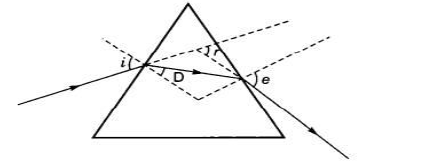a. ∠i and ∠r
b. ∠i and ∠e
c. ∠i, ∠e and ∠D
d. ∠i, ∠r and ∠e

Question : The inability among the elderly to see nearby objects clearly because of the weaking of the ciliary muscles is called-
a. Presbyopia
b. Far sightedness
c. Myopia
d. Near-sightedness
Explanation: As we become old, the power of accommodation of the eye usually decreases, the near point gradually recedes away. This defect is called Presbyopia. Person may suffer from both myopia and hypermetropia.
Correction- Using of Bifocal lens with appropriate power. Bifocal lenses consist of both concave and convex lens, upper position consist of concave lens and lower portion consist of convex lens.

Question : Cone cells helps us to see-
A. In dark
B. In daytime
C. Colours
D. None
a. A and C
b. All of these
c. B and C
d. A and B
Explanation: The photoreceptor cells in the retina are of two types, viz. rod cells and cone cells. The rod cells are sensitive to dim light whereas the cone cells are sensitive to bright light (daytime) and colour.

Question : Colour of eye depends upon colour of which part of eye ?
Answer :  Colour of eye is depends upon the colour of iris.

Question : What is the function of sclerotic in human eye ?
Answer : Sclerotic is to provide a solid shape to eye and protect it from external injuries.

Question : What would have been the colour of the sky, if the Earth had no atmosphere?
Answer : If the earth has no atmosphere, the sky would have appeared black because of no refraction.

Question : What is Tyndall effect? Explain with an example.
Answer :  The scattering of the light by particles, that it encounters in its path, is called Tyndall effect. When a beam of light enters a smoke-filled dark room through a small hole, then its path becomes visible to us. The tiny dust particles present in the air of room scatter the beam of light all around the room. Thus, scattering of light makes the particles visible. Tyndall effect can also be observed when sunlight passes through a canopy of a dense forest, where, the tiny water droplets in the mist scatter the light.

Question : Why is red color selected for danger signal lights?
Answer :  Because the red light has the largest wavelength of all colors, it is the least dispersed. Because of this, much of the light gets reflected back and can travel longer distances through clouds, fog, dust etc. to effectively enter our eyes.

Question : Light of two colors A and B pass through a glass prism. A deviates more than B from its path of incidence. Which color has a higher speed in the prism?
Answer :  Color B has higher speed than that of color A.

Question :  How is the sense of vision carried from the eye to the brain?
Answer :  Optic nerves carry sensation of vision from eye to brain.

Question : State one main function each of iris, pupil, and cornea.
i. Iris controls the size of pupil thus, monitoring the amount of light rays entering through pupil.
ii. Pupil is the aperture through which light enters and reach retina.c
iii. Cornea provides 67% of of eye's focussing power.

Question : Explain with the help of a diagram, how we are able to observe the sunrise about two minutes before the Sun gets above the horizon
The Sun can be seen about two minutes before sunrise because when the Sun is slightly below the horizon, the Sun’s light coming from less dense air to more dense air is refracted downwards as it passes through the atmosphere. Thus due to this atmospheric refraction, the Sun appears to be raised above the horizon when actually it is slightly below the horizon.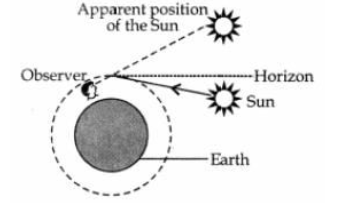Question : i. Why does the power to see near objects as well as far off objects clearly diminish with age? Name the defects that are likely to arise in eye in such a condition.
ii. The far point of a myopic eye is 60 cm. Find the focal length of the lens used to correct it.
Answer :  i. The defects arise due to the gradual weakening of the ciliary muscles which is used to change the shape of lens in eye to help foccusing. Its weaking leads to decreased flexibility of the eye lens. Sometimes, a person may suffer from both myopia and hypermetropia. This condition of defect is called presbyopia. Such people often require bi-focal lenses to correct this defect.

ii. Using lens formula,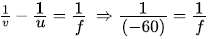[given, v = -60 cm , u = ∞ ]
f = -60 cm. Thus, the lens used will be concave or diverging lens as the focal length is negative.

Question : Explain why the planets do not twinkle but the stars twinkle?
The planets are much closer to the earth. A planet can be considered as a collection of large number of point-sized sources of light. So the total variation in the amount of light entering our eye from all the individual point-sized sources will average out to zero thereby nullifying the twinkling effect.

Question : A student has difficulty in reading the blackboard while sitting in the last row. What could be the defect the child is suffering from? How could it be corrected?
Answer :  The student has difficulty in reading the blackboard in the last row implies that the student has difficulty in seeing the distant objects. This type of defect where a person cannot see beyond certain distance is called myopia. It could be corrected by wearing concave lens of suitable power.

Question : The near point of a hypermetropic eye is 1 m. What is the power of the lens required to correct this defect? Assume that the near point of the normal eye is 25 cm.
Answer : Here, u = - 25 cm (Normal near point)
v = -1 m = -100 cm (Near point of defective eye)
f=?
Using lens formula,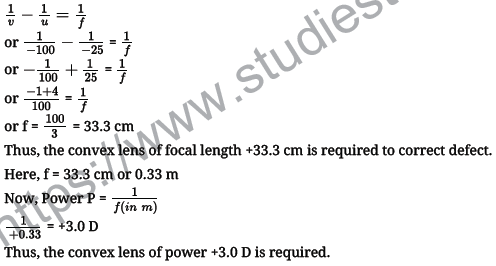Question : i. Demonstrate an activity with a well labelled diagram to prove that white light is made up of seven colours.
ii. Which colour of light bends least and which one the most while passing out from the prism? Also, state the reason for the same.
Answer :  i. The phenomenon of splitting of a beam of white light into its seven constituent colours when passed through a transparent medium is known as dispersion, which was first discovered by Issac Newton in 1666. To understand this phenomenon, let us take a thick sheet of cardboard and make a small hole or narrow slit in its middle allow sunlight to fall on the slit. This gives a narrow beam of white light. Now, take a glass prism and allow this white light to fall one of its faces as shown in figure. Turn the prism slowly until the light that comes out of it appears on a nearby screen.
We see a beautiful band of seven colours on the screen called visible spectrum.

The sequence of colours seen from the lower part of the screen is violet (V), indigo

(I), blue (B), green (G), yellow (Y), orange (O) and red (R). The acronym for this is VIBGYOR.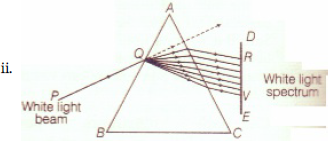Red light has the maximum wavelength and violet light has the minimum wavelength, so in a transparent medium except air and vacuum, red light having the largest wavelength suffers the least deviation while violet light having the least wavelength bends the most.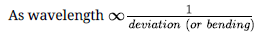CBSE Class 10 Science Chemical Reactions and Equations Assignment
 CBSE Class 10 Science Acids Bases and Salts Assignment Set A CBSE Class 10 Science Acids Bases and Salts Assignment Set B CBSE Class 10 Science Acids Bases and Salts Assignment Set C
 CBSE Class 10 Science Metals and Non Metals Assignment Set A CBSE Class 10 Science Metals and Non Metals Assignment Set B
 CBSE Class 10 Science Carbon and its compounds Assignment Set A CBSE Class 10 Science Carbon and its compounds Assignment Set B CBSE Class 10 Science Carbon and its compounds Assignment Set C CBSE Class 10 Science Carbon and its compounds Assignment Set D CBSE Class 10 Science Carbon and its compounds Assignment Set E
 CBSE Class 10 Science Periodic Classification Of Elements Assignment Set A CBSE Class 10 Science Periodic Classification Of Elements Assignment Set B CBSE Class 10 Science Periodic Classification Of Elements Assignment Set C
 CBSE Class 10 Science Biology Life Process Respiration Assignment CBSE Class 10 Science Biology Nutrition Assignment CBSE Class 10 Science Biology Respiration Assignment CBSE Class 10 Science Biology Transport Enrichment Assignment CBSE Class 10 Science Biology Transportation Assignment CBSE Class 10 Science Life Processes Assignment Set A CBSE Class 10 Science Life Processes Assignment Set B CBSE Class 10 Science Life Processes Assignment Set C
 CBSE Class 10 Science Control and Coordination Assignment Set A CBSE Class 10 Science Control and Coordination Assignment Set B CBSE Class 10 Science Control and Coordination Assignment Set C CBSE Class 10 Science Control and Coordination Assignment Set D
 CBSE Class 10 Science How do Organisms Reproduce Assignment Set A CBSE Class 10 Science How do Organisms Reproduce Assignment Set B
 CBSE Class 10 Science Heredity and Evolution Assignment Set A CBSE Class 10 Science Heredity and Evolution Assignment Set B CBSE Class 10 Science Heredity and Evolution Assignment Set C
 CBSE Class 10 Science Light Reflection and Refraction Assignment Set A CBSE Class 10 Science Light Reflection and Refraction Assignment Set B CBSE Class 10 Science Light Reflection and Refraction Assignment Set C CBSE Class 10 Science Light Reflection and Refraction Assignment Set D CBSE Class 10 Science Light Reflection and Refraction Assignment Set E CBSE Class 10 Science Light Reflection and Refraction Assignment Set F
 CBSE Class 10 Science Human Eye and Colorful World Assignment Set A CBSE Class 10 Science Human Eye and Colorful World Assignment Set B CBSE Class 10 Science Human Eye and Colorful World Assignment Set C
 CBSE Class 10 Physics Electricity Assignment Set A CBSE Class 10 Physics Electricity Assignment Set B CBSE Class 10 Physics Electricity Assignment Set C CBSE Class 10 Science Electricity Assignment Set A CBSE Class 10 Science Electricity Assignment Set B CBSE Class 10 Science Electricity Assignment Set C
 CBSE Class 10 Science Magnetic Effects of Electric Current Assignment Set A CBSE Class 10 Science Magnetic Effects of Electric Current Assignment Set B CBSE Class 10 Science Magnetic Effects of Electric Current Assignment Set C CBSE Class 10 Science Magnetic Effects of Electric Current Assignment Set D
 CBSE Class 10 Science Sources of Energy Assignment Set A CBSE Class 10 Science Sources of Energy Assignment Set B CBSE Class 10 Science Sources of Energy Assignment Set C CBSE Class 10 Science Sources of Energy Assignment Set D
 CBSE Class 10 Science Our Environment Assignment Set A CBSE Class 10 Science Our Environment Assignment Set B CBSE Class 10 Science Our Environment Assignment Set C
 CBSE Class 10 Science Sustainable Management of Natural Resources Assignment Set A CBSE Class 10 Science Sustainable Management of Natural Resources Assignment Set B CBSE Class 10 Science Sustainable Management of Natural Resources Assignment Set C
 CBSE Class 10 Physics Revision Assignment Set A CBSE Class 10 Physics Revision Assignment Set B CBSE Class 10 Physics Revision Assignment Set C CBSE Class 10 Physics Revision Assignment Set D CBSE Class 10 Physics Revision Assignment Set E CBSE Class 10 Physics Revision Assignment Set F CBSE Class 10 Physics Revision Assignment Set G CBSE Class 10 Physics Revision Assignment Set H
 CBSE Class 10 Science Assignment CBSE Class 10 Science Assignments Collection CBSE Class 10 Science Energy Crossword Puzzle Assignment
 CBSE Class 10 Physics Question Bank CBSE Class 10 Physics Tungsten Assignment
 CBSE Class 10 Science Chemistry Cleaning Capacity of Soap Assignment CBSE Class 10 Science Chemistry Determination of pH Assignment CBSE Class 10 Science Chemistry Different Types of Chemical Reactions Assignment CBSE Class 10 Science Chemistry Displacement Reaction and Reactivity Series Assignment CBSE Class 10 Science Chemistry Properties of Acetic Acid Assignment CBSE Class 10 Science Chemistry Saponification Preparation of Soap Assignment CBSE Class 10 Science Chemistry Study of properties of HCI Acid and NaOH Assignment

Tags